# Mathematical induction

(diff) ← Older revision | Latest revision (diff) | Newer revision → (diff)

A method of proving mathematical results based on the principle of mathematical induction: An assertion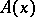, depending on a natural number, is regarded as proved if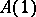has been proved and if for any natural numberthe assumption thatis true implies that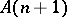is also true.

The proof ofis the first step (or base) of the induction and the proof offrom the assumed truth of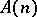is called the induction step. Hereis called the induction parameter and the assumption offor the proof ofis called the induction assumption or induction hypothesis. The principle of mathematical induction is also the basis for inductive definition. The simplest example of such a definition is the definition of the property: "to be a word of length n over a given alphabet a1…ak" .

The base of the induction is: Each symbol of the alphabet is a word of length 1. The induction step is: Ifis a word of length, then each word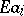, where, is a word of length. Induction can also start at the zero-th step.

It often happens thatandcan be proved by similar arguments. In these cases it is convenient to use the following equivalent form of the principle of mathematical induction. Ifis true and if for each natural numberfrom the assumption:is true for any natural number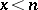, it follows thatis also true for, thenis true for any natural number. In this form the principle of mathematical induction can be applied for proving assertionsin which the parameterruns through any well-ordered set of a transfinite type (transfinite induction). As simple examples of transfinite induction one has induction over a parameter running through the set of all words over a given alphabet with the lexicographic ordering, and induction over the construction formulas in a given logico-mathematical calculus.

Sometimes, for the inductive proof of an assertionone has to inductively prove, simultaneously with, a whole series of other results without which the induction forcannot be carried out. In formal arithmetic one can also give resultsfor which, within the limits of the calculus considered, an induction can not be carried out without the addition of new auxiliary results depending on(see ). In these cases one has to deal with the proof of a number of assertions by compound mathematical induction. All these statements could formally be united into one conjunction; however, in practice this would only complicate the discussion and the possibility of informal sensible references to specific induction assumptions would disappear.

In some concrete mathematical investigations the number of notions and results defined and proved by compound induction has reached three figures (see ). In this case, because of the presence in induction of a large number of cross references to the induction assumptions, for a concise (informal) understanding of any (even very simple) definition or results for a large value of the induction parameter, the reader must be familiar with the content of all induction ideas and properties of these ideas for small values of the induction parameter. Apparently, the only logically correct outcome of this circle of problems is the axiomatic presentation of all these systems of ideas. Thus, a great number of ideas defined by compound mathematical induction lead to the need for an application of the axiomatic method in inductive definitions and proofs. This is a visual example of the necessity of the axiomatic method for the solution of concrete mathematical problems, and not just for questions relating to the foundations of mathematics.

How to Cite This Entry:
Mathematical induction. Encyclopedia of Mathematics. URL: http://encyclopediaofmath.org/index.php?title=Mathematical_induction&oldid=31488
This article was adapted from an original article by S.I. Adyan (originator), which appeared in Encyclopedia of Mathematics - ISBN 1402006098. See original article